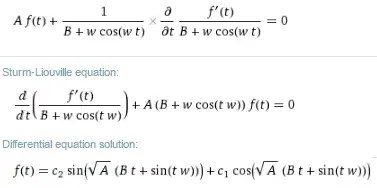# Simplifying Laplace’s Tidal Equations for QBO

[mathjax]I have been playing the models of ENSO and QBO off of each other. So for whatever insights I glean from one model, I have tried to apply it to the other.  My last ENSO analysis involved simplifying Laplace’s Tidal Equations and finding similarity to the Mathieu-modulation used to describe sloshing of water volumes.  However, I had left one equation in a rough state, and stated that I would get back to it.  That’s what I will do in this post, and I can flatly assert that the simplification is an eye-opener for understanding the behavior of QBO! (Which shouldn’t be surprising since Richard Lindzen tried vainly to apply Laplace’s equations to QBO, with the outcome of creating a monster of complexity, see  )

The equation that I had left in an unfinished state was the second-derivative reproduced here:$frac{d^2Theta}{dmu^2} = frac{d^2Theta}{dt^2} frac{d^2t}{dmu^2}$

This is an improper chain rule expansion, which should be:$frac{d^2Theta}{dmu^2} = frac{d}{dt}( frac{dTheta}{dt} frac{dt}{dmu}) frac{dt}{dmu}$

So the simplified Laplace’s equation with no RHS forcing appears as such:$frac{1}{sigma^2} left( frac{d}{dt}(frac{dTheta}{dt} frac{dt}{dmu}) frac{dt}{dmu} + left[frac{s}{sigma} + s^2right] Theta right) + gamma Theta = 0$

For a value of $$frac{dmu}{dt}$$ that is a constant plus one or more sinusoids, this has a solution as a type of Sturm-Liouville equation:So that a possible solution for $$Theta(t)$$ is this$Theta(t) = k cdot sin( sqrt{A} cdot sin(omega t + psi_0) + phi_0)$

This is a strange looking formulation because it is rare to find a sinusoid of a sinusoid in natural phenomena (in contrast to frequent use for FM frequency modulation in engineering comms applications). But this structure is critical for understanding how it applies to the behavior of QBO. I had always asserted that the QBO was composed of sinusoidal components derived from a cyclic lunar gravitational forcing, mainly derived from the nodal or draconic cycling. But this has the undesired effect of creating a modulated beat envelope , which is not observed in the QBO data (which is largely unmodulated). So what the sinusoid of a sinusoid does is gradually clip the extent of the cyclic excursions, with the end result being that the maximal and minimal extents of the composed model are relatively fixed.

Without a lot of fiddling of the model fit, this is a comparison against the QBO 30 hPa time series:This fit makes a lot of physical sense because the $$frac{dmu}{dt}$$ term describes a latitudinal modulation in the Laplace $$Theta$$ formulation, which is exactly what the nodal lunar gravitational force is supplying.  It gets the average fundamental period exactly right, and the slight mix of the other lunar periods generates the modulation in the frequency about the average. The fine structure is provided mainly by higher frequency aliased nodal components.

What also simplifies is the elimination of the RHS forcing. Suggesting that the lunar field was acting as a classical forced response driver seemed tenuous at best, largely because the cumulative mass of the QBO is not that great. However, by invoking the lunar field as a curl force driving the circulation patterns from outside of the equatorial latitude makes much more intuitive sense.

Of course, since ENSO has a much larger mass, the RHS forcing model may still apply there, see this for the late-breaking progress on ENSO. I am only certain that the QBO and ENSO modeling will continue to feed off each other.

Given the good agreement with the QBO model against the data, I still can’t make heads or tails how Richard Lindzen could miss this simplification in the Laplace tidal equations (aka shallow-water wave equations). As I said in the previous post, since the QBO (or ENSO) exists primarily along the equator, most of the complexity disappears as the second-order terms drop out, as does the Coriolis effect.

The other part that Lindzen whiffed badly at was identifying the seasonally-aliased lunar periods as the primary modulation. He even said that if the periods were observed, then it would be difficult to rule it out as a mechanism.Poor Richard, quote taken from 

I may be bucking the conventional wisdom of QBO understanding with this model, but look who is historically responsible for the state of affairs — its the guy with the “deep contrarian streak” — Lindzen. He has been wrong on so many theories, it should not surprise anyone that he took the QBO model way out into left-field and declared premature victory, leaving successive generations of scientists to valiantly try to make sense of it. Fortunately, we can always go back to the source — the genius of Laplace — and work our way forward from his foundational principles.

## References

 R. S. Lindzen, “Planetary waves on beta-planes,” Monthly Weather Review, vol. 95, no. 7, pp. 441–451, 1967.
 R. S. Lindzen and S.-S. Hong, “Effects of mean winds and horizontal temperature gradients on solar and lunar semidiurnal tides in the atmosphere,” Journal of the atmospheric sciences, vol. 31, no. 5, pp. 1421–1446, 1974.

## 6 thoughts on “Simplifying Laplace’s Tidal Equations for QBO”

1.Keith Pickering

Not only would I encourage you to publish this, but to include in your publication predictions of QBO for 20 to 50 years in advance. That is the only way to distinguish your work from some of the other nonsense that has been making the rounds lately.

Like

•admin

Thanks Keith, a possible paper is either going in Physical Review Letters or nowhere. I don’t hold up high hopes for PRL though.

Like

2.admin

Got another AGW-denying atmospheric scientist to comment on the QBO model

So Spencer also appears to claim that the QBO period is an emergent property of their model, with no external forcing to drive it.

Like